# Algebraic polynomial of best approximation

Jump to: navigation, search

A polynomial deviating least from a given function. More precisely, let a measurable function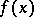be in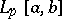(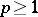) and let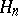be the set of algebraic polynomials of degree not exceeding. The quantity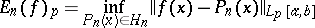(*)

is called the best approximation, while a polynomial for which the infimum is attained is known as an algebraic polynomial of best approximation in. Polynomials which deviate least from a given continuous function in the uniform metric (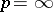) were first encountered in the studies of P.L. Chebyshev (1852), who continued to study them in 1856 . The existence of algebraic polynomials of best approximation was established by E. Borel . Chebyshev proved that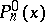is an algebraic polynomial of best approximation in the uniform metric if and only if Chebyshev alternation occurs in the difference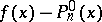; in this case such a polynomial is unique. If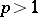, the algebraic polynomial of best approximation is unique due to the strict convexity of the space. If, it is not unique, but it has been shown by D. Jackson  to be unique for continuous functions. The rate of convergence of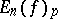to zero is given by Jackson's theorems (cf. Jackson theorem).

In a manner similar to (*) an algebraic polynomial of best approximation is defined for functions in a large number of unknowns, say. If the number of variables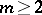, an algebraic polynomial of best approximation in the uniform metric is, in general, not unique.